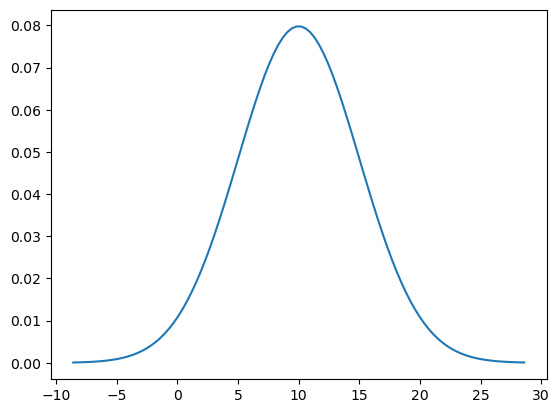# How to plot continuous probability distributions (in Python, using SciPy)

See all solutions.

There are many famous continuous probability distributions, such as the normal and exponential distributions. How can we get access to them in software, to plot the distribution as a curve?

## Solution

You can import many different random variables from SciPy’s stats module. The full list of them is online here.

The challenge with plotting a random variable is knowing the appropriate sample space, because some random variables have sample spaces of infinite width, which cannot be plotted.

But we can just ask SciPy to show us the central 99.98% of a continuous distribution, which is almost always indistinguishable to the human eye from the entire distribution.

We style the plot below so that it is clear the sample space is continuous.

1
2
3
4
5
6
7
8
9
10
11
from scipy import stats
X = stats.norm( 10, 5 )      # use a normal distribution with μ=10 and σ=5

xmin = X.ppf( 0.0001 )       # compute min x as the 0.0001 quantile
xmax = X.ppf( 0.9999 )       # compute max x as the 0.9999 quantile
import numpy as np
xs = np.linspace( xmin, xmax, 100 )  # create 100 x values in that range

import matplotlib.pyplot as plt
plt.plot( xs, X.pdf( xs ) )  # plot the shape of the distribution
plt.show()